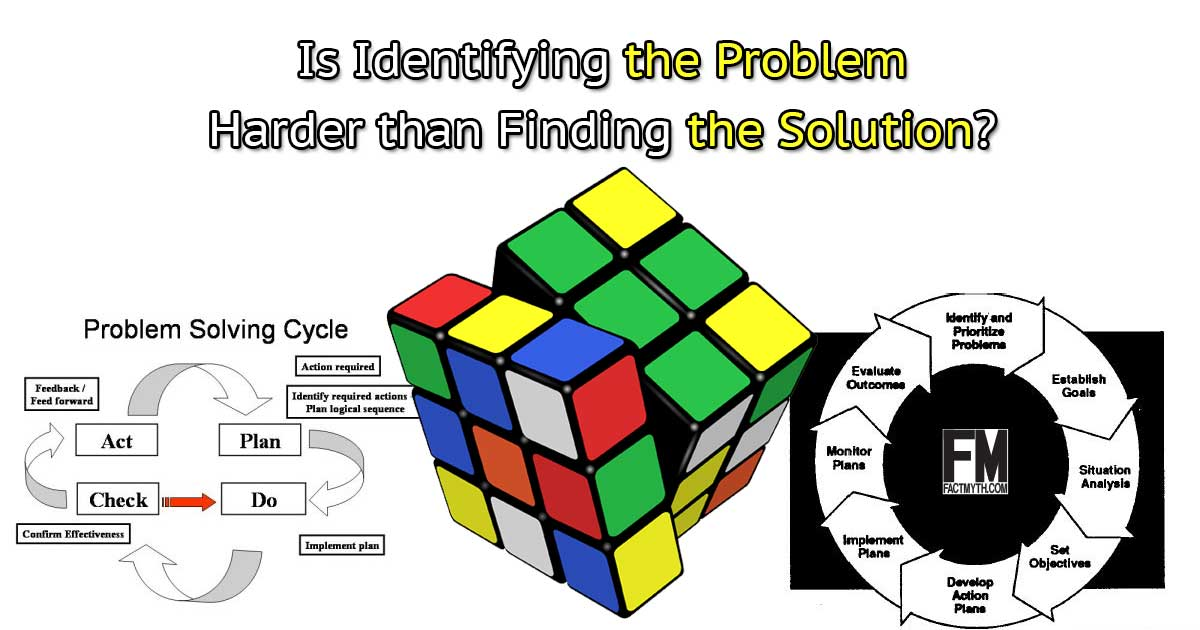# Identifying a Problem is Often Harder than Solving it fact## Is it Easier to Identify a Problem or to Solve a Problem?

Identifying a problem is often harder than solving it. Solving a problem requires a proper diagnosis, and that requires asking the right questions. With a properly worded question, many solutions become clear. While this logic doesn’t apply to every situation, it does seem to work as a rule of thumb.

Albert Einstein’s Secret and How He Solved The World’s Hardest Problems. Before he could solve a problem, he had to ask the right questions.

FACT: Einstein is quoted as having said that if he had one hour to save the world, he would spend fifty-five minutes defining the problem and only five minutes finding the solution.

## Definitions of Problem-Solving Terms

To understand the concept above, let’s do a quick overview of some terms.

• Problem-Solving: The process of working through details of a problem to reach a solution. This requires figuring out what the problem is first.
• Diagnosis in problem-solving: is the identification of the nature and cause of a certain phenomenon.
• A solution in problem-solving: is finding the correct answer to a problem.

## Examples of Problem-Solving and Diagnosis

An example of this is a doctor detecting cancer; our intuition tells us that detecting the cancer is easy, and treating it is hard. This can be true but think about it another way. If we can laser focus our diagnosis, and be sure of the root causes and locations of cancer, we likely already have the medical knowledge to treat it. In other words, the better we can diagnose the issue, the easier the solution becomes. This is what is meant that “the problem is knowing what the problem is” or “diagnosing a problem is often more difficult than solving it.”

Now let’s consider social justice. We may think it is easy to identify injustice in society, and understanding how to “fix” it is more complicated. However, again, we are over-simplifying the concept of “problem” in that thought process. If we truly spot the areas of life that are causing the injustice, a solution becomes apparent; when a question is phrased improperly, the solution seems harder.

For instance, when considering economic inequality, we could say, “the problem is a low velocity of money leading to income inequality.” However, instead we might say, “the root problem seems to be politicians taking funding from special interests, which leads to loopholes, which allow for a shadow economy that contributes to the low velocity and leads to wealth inequality over time.” This brings us to the question of “how can we address this without sacrificing liberty?” Or we may need to dig even deeper. By asking more accurate questions, we find better answers and make our way toward the root problem. Only by addressing the cause of symptoms can problems be solved, even if the final solution ends up being one of dealing with the symptoms, as it would be with a common cold.

We can also apply this logic to science. We may find a problem in physics, like Newton, and ask, “why do things fall toward earth?” It seems like a solution is much harder than the question, however, Einstein found the solution only after he had spent years of questioning the nature of light, space, and time and examining earlier solutions. After Einstein finally had his “ah-ha” moment, the actual solution became clear “velocity and mass cause spacetime to curve around objects with mass.” (see Einstein’s Pathway to General Relativity.)

The simplest example is an electronic device. Diagnosing the problem is sometimes nearly impossible, but the common solution “turning it off and on again” isn’t.

Instagram Co-founder Kevin Systrom: Finding the Problem is the Hard Part.

TIP: This line of reasoning falls apart if you consider diagnosis as part of solving a problem. We want to be clear that we are comparing the process of figuring out the right question and diagnosis, to the actual art of implementing the solution.

## Why is Understanding this Important?

This concept is somewhat semantic, but it is meant as a reminder finding the right solution is all about asking the right questions.

We can look around in society and see inequality as a broad concept, but addressing inequality in a way that actually “fixes” the problem requires a proper diagnosis, and a proper diagnosis requires asking the right questions and truly understanding the problem.

Working backward to solve problems – Maurice Ashley. A video comparing problem-solving and game theory to chess.

FACT: The scientific method is all about finding a falsifiable hypothesis based on an observation that has predictive power, and then putting it to the test through experiment. The key to a good theory is asking the right questions.

1. Theory of Problem Solving” Sciencedirect.com
2. Einstein’s Secret to Amazing Problem Solving (and 10 Specific Ways You Can Use It)” Litemind.com